# Eight palm

There grows 8 palms by the sea. At the first sitting one parrot, on second two, on third sits four parrots on each other twice the previous parrots sitting on a previous palm. How many parrots sitting on eighth palm?

n =  128

### Step-by-step explanation:

$n={2}^{8-1}=128$We will be pleased if You send us any improvements to this math problem. Thank you!## Related math problems and questions:

• Gp - 80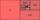One of the first four members of a geometric progression is 80. Find its if we know that the fourth member is nine times greater than the second.
• LoanApply for a \$ 59000 loan, the loan repayment period is 8 years, the interest rate 7%. How much should I pay for every month (or every year if paid yearly). Example is for practise geometric progression and/or periodic payment for an annuity.
• Quotient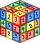Determine the quotient and the second member of the geometric progression where a3=10, a1+a2=-1,6 a1-a2=2,4.
• Geometric progressionIn geometric progression, a1 = 7, q = 5. Find the condition for n to sum first n members is: sn≤217.
• Geometric progression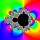Fill 4 numbers between 4 and -12500 to form geometric progression.
• Students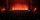In the front row sitting three students and in every other row 11 students more than the previous row. Determine how many students are in the room when the room is 9 lines, and determine how many students are in the seventh row.
• GP - 8 itemsDetermine the first eight members of a geometric progression if a9=512, q=2
• Piano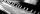If Suzan practicing 10 minutes at Monday; every other day she wants to practice 2 times as much as the previous day, how many hours and minutes will have to practice on Friday?
• Progression-12, 60, -300,1500 need next 2 numbers of pattern
• Miraculous treeMiraculous tree grows so fast that the first day increases its height by half the total height of the second day by the third, the third day by a quarter, etc. How many times will increase its height after 6 days?
• Q of GP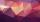Calculate quotient of geometric progression if a1=5 a a1+a2=12.
• Wire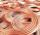One pull of wire is reduced its diameter by 14%. What will be the diameter of wire with diameter 19 mm over 10 pulls?
• SaleIf the product twice price cut by 25%, what percentage was price cut in total?
• Geometric progressiobIf the sum of four consective terms of geometric progression is 80 and arithmetic mean of second and fourth term is 30 then find terms?
• Ray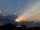Light ray loses 1/19 of brightness passing through glass plate. What is the brightness of the ray after passing through 7 identical plates?
• Three members GPThe sum of three numbers in GP (geometric progression) is 21 and the sum of their squares is 189. Find the numbers.
• CrystalThe crystal grows every month 1.2 permille of its mass. For how many months to grow a crystal from weight 177 g to 384 g?